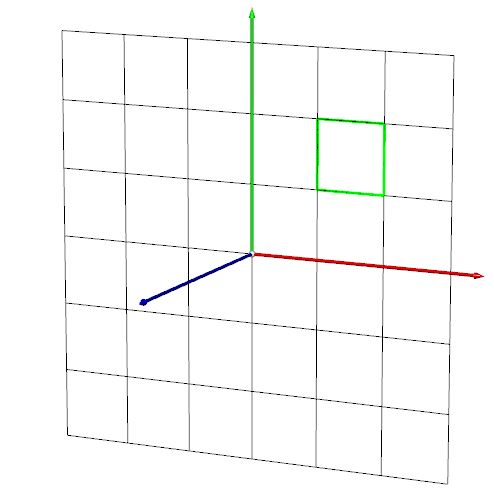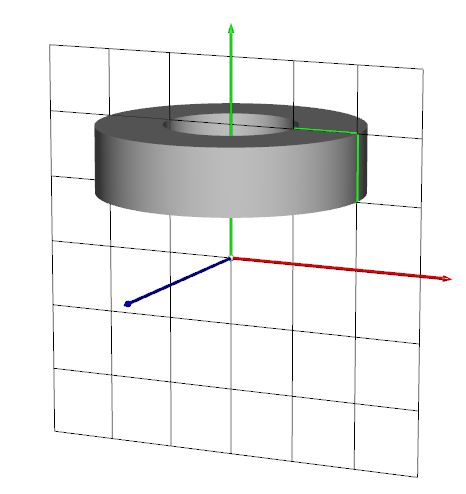Surface of Revolution is a simple process to create new geometry. Spheres, cylinders, cones, and torus, all can be created using this approach. This library provides the functionality required to build such geometry. Some geometry is already prebuilt as sohwn in the image below.In order to create a surface of revolution we need a 2D profile, i.e., a curve or set of line segments, and an axis about which the profile will revolve. For instance, considering the chess pawn presented in the figure above, the profile is as presented in the image below. In here the axis is always the Y axis.the 2D profile is a set of points in the XY plane. For instance consider the following profile (green line on the left) and associated surface of revolution.Codewise this surface can be defined with the following function:

`void create (float *p, int numP, int sides, int closed, float smoothCos);`

The function receives an array of floats `p` where the points are specified in 2D (XY plane); `numP` provides the number of points; `sides` determines the number of sides in the figure, the greater the number the smoother the surface; `closed` is a flag indicating if the 2D profile is closed or not, if closed then the first point is added to the end of the list; `smoothCos` parameter is used to preserve edges, all edges with a cosine (in radians) larger than the value provided will be smoothed (note the hard edges on the top and bottom of the above figure, and the smooth lateral edges).

The example surface above can be defined as:

```VSSurfRevLib mySurfRev;
...
float p1[] = { 1,2,     1,1,    2,1,    2,2};
mySurfRev.create(p1, 4, 4096, 1, 1.0f);
```

A set of functions is also provided to generate common geometric shapes:

```